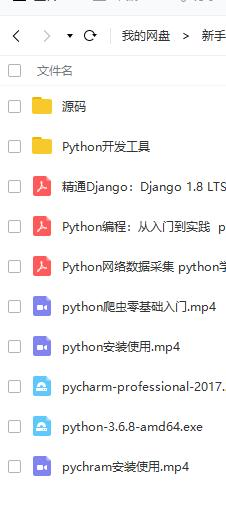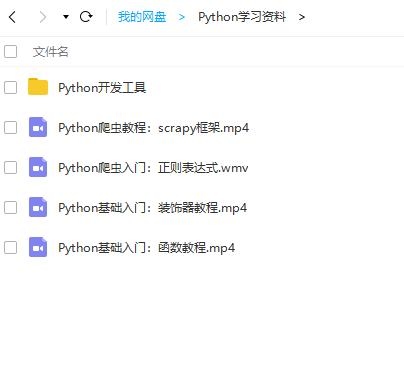### python超实用的30 个简短的代码片段（三）

Python是目前最流行的语言之一，它在数据科学、机器学习、web开发、脚本编写、自动化方面被许多人广泛使用。

PS：如有需要Python学习资料的小伙伴可以加点击下方链接自行获取http://t.cn/A6Zvjdun

## 21.使用枚举

```list = ["a", "b", "c", "d"]
for index, element in enumerate(list):
print("Value", element, "Index ", index, )
# ('Value', 'a', 'Index ', 0)
# ('Value', 'b', 'Index ', 1)
#('Value', 'c', 'Index ', 2)
# ('Value', 'd', 'Index ', 3)```

## 22.计算所需时间

```import time
start_time = time.time()
a = 1
b = 2
c = a + b
print(c) #3
end_time = time.time()
total_time = end_time - start_time
print("Time: ", total_time)
# ('Time: ', 1.1205673217773438e-05)```

## 23.Try else 指令

```try:
2*3
except TypeError:
print("An exception was raised")
else:
print("Thank God, no exceptions were raised.")
#Thank God, no exceptions were raised.```

## 24.查找最常见元素

```def most_frequent(list):
return max(set(list), key = list.count)

list = [1,2,1,2,3,2,1,4,2]
most_frequent(list)```

## 25.回文

```def palindrome(string):
from re import sub
s = sub('[W_]', '', string.lower())
return s == s[::-1]
palindrome('taco cat') # True```

## 26.没有 if-else 语句的简单计算器

```import operator
action = {
"-": operator.sub,
"/": operator.truediv,
"*": operator.mul,
"**": pow
}
print(action['-'](50, 25)) # 25```

## 27.元素顺序打乱

```from copy import deepcopy
from random import randint
def shuffle(lst):
temp_lst = deepcopy(lst)
m = len(temp_lst)
while (m):
m -= 1
i = randint(0, m)
temp_lst[m], temp_lst[i] = temp_lst[i], temp_lst[m]
return temp_lst

foo = [1,2,3]
shuffle(foo) # [2,3,1] , foo = [1,2,3]```

## 28.列表扁平化

```def spread(arg):
ret = []
for i in arg:
if isinstance(i, list):
ret.extend(i)
else:
ret.append(i)
return ret

## 29.变量交换

```def swap(a, b):
return b, a
a, b = -1, 14
swap(a, b) # (14, -1)```

## 30.获取缺失键的默认值

```d = {'a': 1, 'b': 2}
print(d.get('c', 3)) # 3```

# 2020年最新Python教程：